Next: Outer Product Up: Fundamental Concepts Previous: Bra Space

# Operators

We have seen that a functional is a machine that inputs a ket and spits out a complex number. Consider a somewhat different machine that inputs a ket and spits out another ket in a deterministic fashion. Mathematicians call such a machine an operator. We are only interested in operators that preserve the linear dependencies of the kets upon which they act. Such operators are termed linear operators. Consider an operator labeled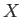. Suppose that when this operator acts on a general ket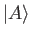it spits out a new ket which is denoted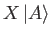. Operatoris linear provided that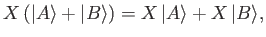(1.26)

for all ketsand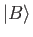, and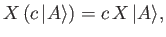(1.27)

for all complex numbers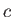. Operatorsand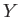are said to be equal if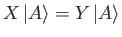(1.28)

for all kets in the ket space in question. Operatoris termed the null operator if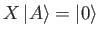(1.29)

for all kets in the space. This operator is usually denoted 0 . It follows from Equation (1.5) that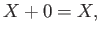(1.30)

whereis a general operator. Operatoris termed the identity operator if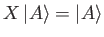(1.31)

for all kets in the space. This operator is usually denoted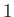. Operators can be added together. Such addition is defined to obey a commutative and associate algebra: that is,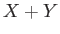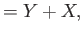(1.32)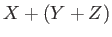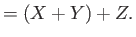(1.33)

Operators can also be multiplied. Operator multiplication is associative: that is,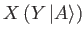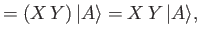(1.34)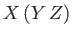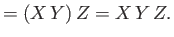(1.35)

However, in general, operator multiplication is non-commutative: that is,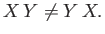(1.36)

So far, we have only considered linear operators acting on kets. We can also give a meaning to their operation on bras. Consider the inner product of a general bra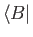with the ket. This product is a number that depends linearly on. Thus, it may be considered to be the inner product ofwith some bra. This bra depends linearly on, so we may look on it as the result of some linear operator applied to. This operator is uniquely determined by the original operator, so we might as well call it the same operator acting on. A suitable notation to use for the resulting bra whenoperates onis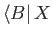. The equation which defines this vector is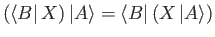(1.37)

for anyand. The triple product of,, andcan be written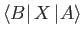without ambiguity, provided we adopt the convention that the bra always goes on the left, the operator in the middle, and the ket on the right.

Consider the dual bra to. This bra depends antilinearly on(i.e., ifis multiplied by the complex numberthen the corresponding bra is multiplied by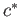) and must therefore depend linearly on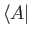. Thus, it may be regarded as the result of some linear operator applied to. This operator is termed the adjoint of, and is denoted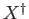. Thus,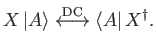(1.38)

It is readily demonstrated that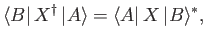(1.39)

plus(1.40)

It is also easily seen that the adjoint of the adjoint of a linear operator is equivalent to the original operator. (See Exercise 4.) An Hermitian operator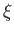has the special property that it is its own adjoint: that is,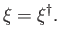(1.41)

Obviously, a complex number can be regarded as a trivial operator that modifies the length and phase of a ket upon which it acts, without changing the ket's direction. Furthermore, it follows from Equations (1.17) and (1.27) that a complex number operator,, commutes with any other operator, and that its adjoint is. Finally, it is easily appreciated that the identity operator corresponds to the number unity, while the null operator corresponds to the number zero.Next: Outer Product Up: Fundamental Concepts Previous: Bra Space
Richard Fitzpatrick 2016-01-22